Courses

# Shear Stresses In Beams Civil Engineering (CE) Notes | EduRev

## Civil Engineering (CE) : Shear Stresses In Beams Civil Engineering (CE) Notes | EduRev

The document Shear Stresses In Beams Civil Engineering (CE) Notes | EduRev is a part of the Civil Engineering (CE) Course Civil Engineering SSC JE (Technical).
All you need of Civil Engineering (CE) at this link: Civil Engineering (CE)

Shear Stresses In Beams

SHEAR STRESS DISTRIBUTION

(i) Rectangular Beam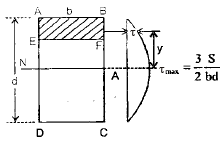• The intensity of shear at a fiber on the plane of cross-section located.the level of EF at a distance y form the neutral axis is given by,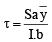Where S = SF at the section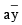= moment of the area above
EF y = distance form neutral axis.
I = moment of inertia about N.A.
b = width of the beam at the level EF 

• Shear stress in terms of y form N.A. is given by•  Average shear stress is given by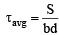• Maximum shear stress occurs at the N.A. and is given by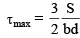Hence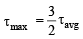• The distance from N.A. at which the average shear  stress is equal to the local shear stress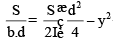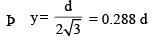(ii) Solid Circular Section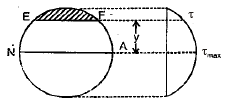• The shear stress at a fibre on the plane of cross-section located at a distance y from neutral axis is given by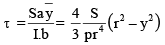• Maximum shear stress  occurs at the N.A. & is given by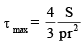• Average shear stress is given by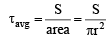Hence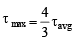• The distance from N.A. at which the local shear stress is equal to average shear stress is given by t avg= t local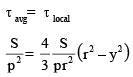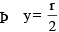(iii) Triangular Section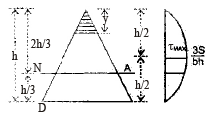• Shear stress at a distance y form vortex is given by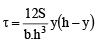• Maximum shear stress exists at y = h/2 (at the middle of triangle) and is given by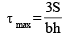• Average shear stress is given by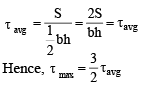• Shear stress at N.A.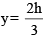is given by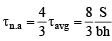(iv) Diamond Section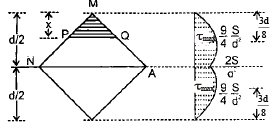• Shear stress at level PQ is given by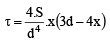• Shear stress at N.A.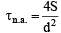• Average shear stress =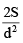Hence ζn.a. = ζavg

• Maximum shear stress occurs at 3/8 d form top and bottom or d/8 form neutral axis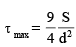Hence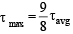(V) I Section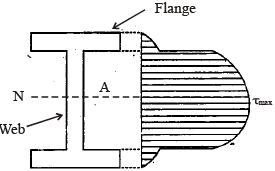• Shear Stress distribution in flange: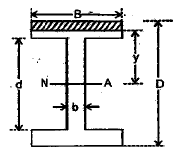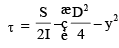• Shear stress at the junction of flange & web, but  within the flange.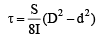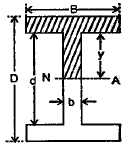• Shear stress distribution within the web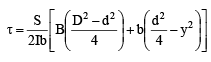• Maximum shear stress exists at N.A. and is given by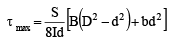• Shear stress at the junction of web and flange but within the web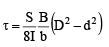• Shear stress distribution in some other  section: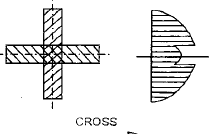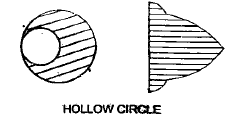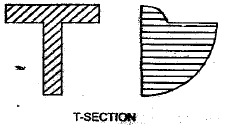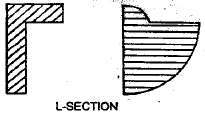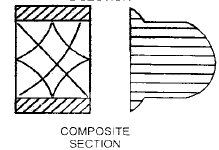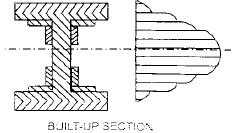CORE OF SECTIONS OF DIFFERENT SHAPES

1. Rectangular Section In order that tension may not develop, we have the condition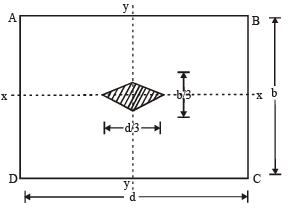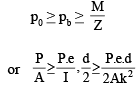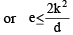wherek = radius of gyration of the section with respect to the NA

d = depth of the section

Thus, for not tension in the section, the eccentricity must not exceed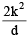.
For a rectangular section of width b and depth d.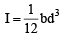and A = b.d.
Hence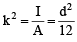Substituting this value of k, we get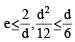or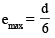Thus the stress will be wholly compressive throughout the section, if the line of action of P falls within the rhombus (as shaded portion of figure), the diagonals of which are of length d/3 and b/3 respectively. This rhombus is called the core or kern of the rectangular section.

2. Solid Circular Section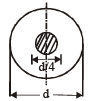The core of a solid circular section is a circle, with the same centre, and diameter d/4.

3. Hollow Circular Section

For a hollow circular section,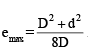Hence the core for a hollow circular section is a concentric circle of diameter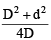where d = inner diameter, D = outer diameter.

Offer running on EduRev: Apply code STAYHOME200 to get INR 200 off on our premium plan EduRev Infinity!

## Civil Engineering SSC JE (Technical)

113 docs|50 tests

,

,

,

,

,

,

,

,

,

,

,

,

,

,

,

,

,

,

,

,

,

;